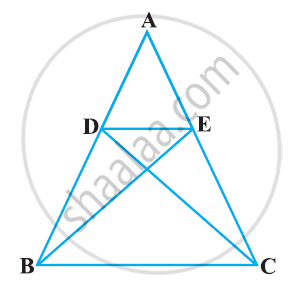Share

# In the following figure, if ΔABE ≅ ΔACD, show that ΔADE ∼ ΔABC. - CBSE Class 10 - Mathematics

ConceptCriteria for Similarity of Triangles

#### Question

In the following figure, if ΔABE ≅ ΔACD, show that ΔADE ∼ ΔABC.#### Solution

It is given that ΔABE ≅ ΔACD.

∴ AB = AC [By CPCT] (1)

And, AD = AE [By CPCT] (2)

(AD)/(AB) = (AE)/(AC)   [Dividing equation 2 by 1]

A = ∠A [Common angle]

∴ ΔADE ∼ ΔABC [By SAS similarity criterion]

Is there an error in this question or solution?

#### APPEARS IN

NCERT Solution for Mathematics Textbook for Class 10 (2019 to Current)
Chapter 6: Triangles
Ex. 6.30 | Q: 6 | Page no. 140

#### Video TutorialsVIEW ALL 

Solution In the following figure, if ΔABE ≅ ΔACD, show that ΔADE ∼ ΔABC. Concept: Criteria for Similarity of Triangles.
S